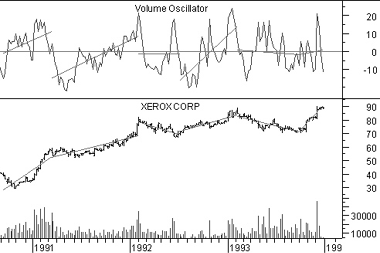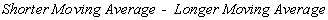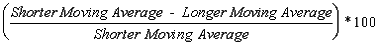Technical Analysis from A to Z

by Steven B. Achelis

VOLUME OSCILLATOR

Overview

The Volume Oscillator displays the difference between two moving averages of a security's volume. The difference between the moving averages can be expressed in either points or percentages.

Interpretation

You can use the difference between two moving averages of volume to determine if the overall volume trend is increasing or decreasing. When the Volume Oscillator rises above zero, it signifies that the shorter-term volume moving average has risen above the longer-term volume moving average, and thus, that the short-term volume trend is higher (i.e., more volume) than the longer-term volume trend.

There are many ways to interpret changes in volume trends. One common belief is that rising prices coupled with increased volume, and falling prices coupled with decreased volume, is bullish. Conversely, if volume increases when prices fall, and volume decreases when prices rise, the market is showing signs of underlying weakness.

The theory behind this is straight forward. Rising prices coupled with increased volume signifies increased upside participation (more buyers) that should lead to a continued move. Conversely, falling prices coupled with increased volume (more sellers) signifies decreased upside participation.

Example

The following chart shows Xerox and 5/10-week Volume Oscillator.I drew linear regression trendlines on both the prices and the Volume Oscillator.

This chart shows a healthy pattern. When prices were moving higher, as shown by rising linear regression trendlines, the Volume Oscillator was also rising. When prices were falling, the Volume Oscillator was also falling.

Calculation

The Volume Oscillator can display the difference between the two moving averages as either points or percentages. To see the difference in points, subtract the longer-term moving average of volume from the shorter-term moving average of volume:To display the difference between the moving averages in percentages, divide the difference between the two moving averages by the shorter-term moving average:This online edition of Technical Analysis from A to Z is reproduced here with permission from the author and publisher.